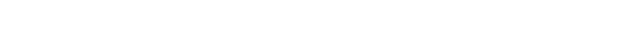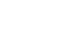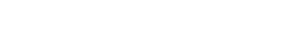A reliable broker, online signals and a robot!
Better together than separately!

In the world economy, there is a clear trend towards globalization, in connection with which it became necessary to compare the potentials of different states. Since most macroeconomic indicators are calculated in the currencies of certain countries, economists are faced with the problem of converting money. GDP is one of the most important indicators of the economic condition of a particular region or state as a whole. To calculate it, the coefficients for comparing the purchasing power of a particular currency are used, determined by the ratio of the cost of a set of similar goods in different countries (shopping basket).

So, we come to the term "purchasing power parity", which I want to talk about in this article. After reading it, you will learn what PPP is, how it is calculated, how it is applied Forex traders and what is the role of parity in international business.

## Essence of purchasing power parity (PPP)

PPP - an economic term that defines the principle of establishing the exchange rate based on the changing cost of similar goods in two states. Originally conceived as a way of assessing long-term equilibrium exchange rates, today it is used to conduct economic analysis in various countries. The modern concept was proposed by Kassel in the early 20th century. It is based on the concept of the purchasing power of a currency, that is, the ability of money to be exchanged for goods or services (goods).

For a better understanding, consider an example. Let's say a certain set of products in the Russian Federation costs 1000 rubles, and an identical set in the USA costs 50 dollars. To determine the fair exchange rate, you need to divide the amount spent on the purchase of these products in the Russian Federation by their price in the USA: 1000/50 = 20. As a result, we get 20 rubles for 1 dollar. With different quotations, the profit for the sale of products in another country would be zero. For example, if the exchange rate was 30 rubles per \$ 1, traders would be able to make a profit of 5 rubles per unit. Ultimately, either of two possible events must happen: the prices of the products equalize, or the exchange rate will be adjusted.

Ideal trading conditions are rare in today's world. For this reason, I made the following conclusion: purchasing power parity is a theoretical concept that is a benchmark for changes in quotations in the long term. The simplest way to calculate the "real" exchange rate at PPP is Big Mac index, introduced by representatives of The Economist magazine in 1986.

## Purchasing Power Parity - Calculation Formula

As mentioned above, PPP is such a ratio of two compared currencies, in which for a certain amount of money (expressed in these currencies) you can buy an equal (in quality, quantity and other criteria) product in two compared countries. Based on this definition, we can derive a formula for purchasing power parity:

PPP = P1 / P2, where:

• PPP - purchasing power parity, showing the "real" exchange rate in a particular country;
• P1 is the cost of the product or service in the first country;
• P2 is the cost of a similar product or service in a comparable country.

The UN conducts international comparisons to determine PPPs using up to 800 different consumer goods and services, as well as about 300 investment goods and up to 20 real estate properties. PPP is calculated as follows:

• Determine the value of the above goods and objects in the currency of one country;
• Determine the prices of the same goods and objects in the United States;
• The PPP is calculated as the quotient of dividing the value in national currency and the price in the United States for each position.

This approach makes it possible to give a more objective assessment of the real exchange rate of a particular state.

## Absolute and relative PPP

The absolute PPP demonstrates the equilibrium of exchange rates at the same cost of the consumer basket in the two compared countries. PPP theory suggests that in the event of inequality of national baskets, the exchange rate will change under the influence of market forces.

Let's say we need to compare two countries (St1 is the cost of a basket in the first country, St2 is the cost of a similar basket in the second), and the exchange rate is represented by the Exc parameter. As a result, we get the following dependence:

St1 = St2 * Exc

So, the course on PPP theory is equal to the ratio of the cost of a set of goods (services) in one country to the cost of similar goods (services) in another. If the baskets are not identical, this rule does not work. How to calculate the "real" rate if it is not possible to find identical baskets of goods? In this case, the relative PPP is used.

The relative PPP is considered as a dynamic version of the absolute PPP, taking into account the rate of inflation and the movement of exchange rates over certain periods of time.

Let's say country A uses currency A, and country B uses B. The value of a basket of goods (P) in country A is PA, and the value of the same basket of goods (Q) in country B is C * PA, where C is unchanged in time value. Alternatively, the value of a basket of goods in country B can be expressed as the product of C * P * SB, where S is the amount of currency of country B (variable) that must be spent to buy one currency of country A. That is, S is the actual exchange rate.

If we assume that (1) and (2) correspond to different dates, we get the following dependence:Based on this formula, we will find the ratio of rates for different periods:After some transformations, we get the following:In simple terms, to determine the rate of decline in the nominal exchange rate, you need to find the difference between the inflation rates in the two countries. Since absolute parity is possible only when C = 1, it is a special case of relative PPP.

## Forex parity, disadvantages of PPP theory

I, like any other car enthusiast, noticed that gasoline prices differ in different cities: the law of a single price does not work even within one country. This is due to various obstacles to free trade. First, we live in a world with non-zero transportation costs. Secondly, data on the cost of goods and services are freely available. In this regard, differences in value arise, which are canceled during the revaluation process. Tariffs and fees are an additional obstacle to international trade.

We must not forget about the effect of non-trading costs. For example, labor and land also have their own value, but they are not included in price baskets, since they cannot be sold. Another drawback of the PPP theory is that it assumes perfect competition in the market.

Traders use purchasing power parity as a rough guide to the FX rate (whether overpriced or undervalued). Try it yourself based on the above formulas, calculate PPP and evaluate the FX rate. You will come to the conclusion that it is advisable to use it for long-term investment, and most CDF traders take into account short-term timeframes (day, week).

## Role of teaching staff in international business

Purchasing power parity is most often used to compare wages and GDP levels in different countries. In terms of GDP, parity allows one to estimate the cost of a product in the compared countries, taking into account the real cost of living of their citizens.

For example, you need to compare the GDP of the Russian Federation and the United States. The easiest way is to determine the USD / RUB ratio and convert the RF GDP to a value expressed in US dollars. The result obtained does not correspond to reality due to several factors:

1. The movements of financial flows in these countries are not taken into account;
2. Currency fluctuations are not taken into account;
3. The relationship between prices and the real value of the currency is not taken into account.

The GDP of one state can suddenly increase relative to another as a result of a fall in the exchange rate. PPP rates are characterized by higher stability in comparison with market exchange rates, and allow a more accurate assessment of the standard of living in different countries. Nominal GDP (Nominal), as well as calculated on the basis of PPP (PPP) for each country in the world, is presented in the figure below.## Conclusions

PPP is an indicator used by economists to compare living standards across countries. While being a good benchmark for long-term equilibrium rates, PPPs are unlikely to be used as a reliable tool for short-term forecasting. Nevertheless, any trader (even if he focuses on short-term trading) will appreciate the essence of purchasing power parity., to track long-term market trends. If you still have questions, the video contains comprehensive information:

Recommended